# Class 8 Maths NCERT Solutions for Chapter – 2 Linear Equations In One Variable Ex – 2.2

### Linear Equations In One Variable

Question 1.
If you subtract$\frac { 1 }{ 2 }$ from a number and multiply the result$\frac { 1 }{ 2 }$ by you get$\frac { 1 }{ 8 }$ What is the number ?

Solution.
Let the required number be x. Then,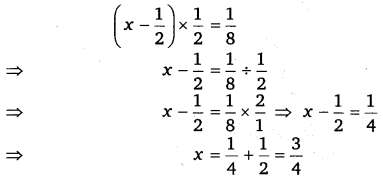Thus, the required number is$\frac { 3 }{ 4 }$

Question 2.
The perimeter of a rectangular swimming pool is 154 m. Its length is 2 m more than twice its breadth. What are the length and the breadth of the pool ?

Solution.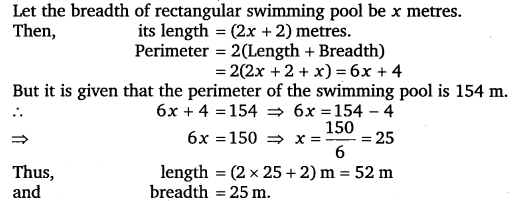Question 3.
The base of an isosceles triangle is$\frac { 4 }{ 3 }$cm. The perimeter of the triangle is$4\frac { 2 }{ 15 }$cm. What is the length of either of the remaining equal sides ?

Solution.
Let each equal side of an isosceles triangle ABC be x cm.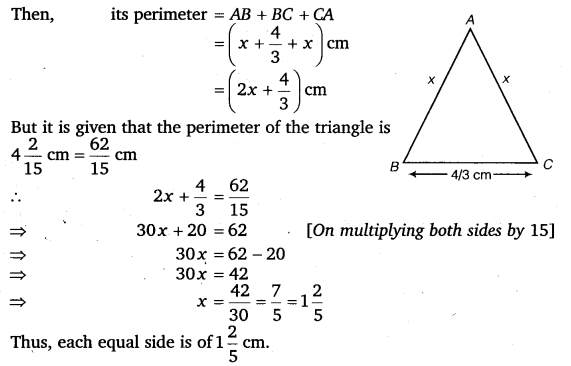Question 4.
Sum of two numbers is 95. If one exceeds the other by 15, find the numbers.

Solution.
Let the number be x and (x + 15).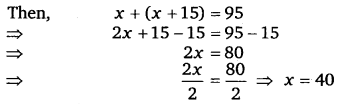Hence, the numbers are 40 and 55.

Question 5.
Two numbers are in the ratio 5 :3. If they differ by 18, what are the numbers ?

Solution.
Let the number br 5x and 3x. Then,Hence, the numbers are 45 and 27.

Question 6.
Three consecutive integers add up to 51. What are these integers ?

Solution.
Let the consecutive numbers be x, (x + 1) and (x + 2). Then,Hence, the consecutive numbers are 16, 17 and 18.

Question 7.
The sum of three consecutive multiples of 8 is 888. Find the multiples.

Solution.
Let the three consecutive multiples of 8 be 8x, 8(x + 1) and 8(x + 2).Hence, the three consecutive multiples of 8 are 8 x 36, 8 x (36 + 1) and 8 x (36 + 2) i.e., 288, 296 and 3.4.

Question 8.
Three consecutive integers are such that when they are taken in increasing order and multiplied by 2, 3 and 4 respectively, they add up to 74. Find these numbers.

Solution.
let the three consecutive integers be x, (x + 1) and (x + 2). It is given that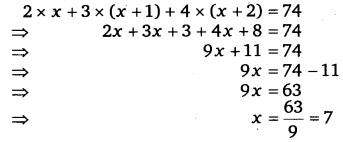Hence, the numbers are 7, 8 and 9

Question 9.
The ages of Rahul and Haroon are in the ratio 5 : 7. Four years later, the sum of their ages will be 56 years. What are their present ages ?

Solution.
Let the ages of Rahul and haroon be 5x and 7x years.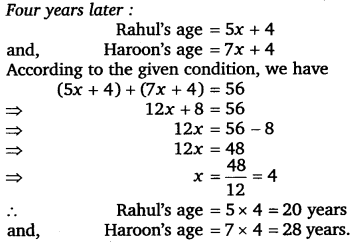Question 10.
The numbers of boys and girls in a class are in the ratio 7 : 5. The number of boys is 8 more than the number of girls. What is the total class strength ?

Solution..
Let the number of boys and girls in a class be 7x and 5x.
Since the number of boys is 8 more than the number of girls. Therefore,
7x = 5x + 8
⇒   7x – 5x = 8
⇒  2x = 8
⇒    x = 4
∴ The number of boys = 7 x 4 = 28
and the number of girls = 5 x 4 = 20
Thus, the total strength of the class is 28 + 20, i. e., 48.

Question 11.
Baichung’s father is 26 years younger than Baichung’s grandfather and 29 years older than Baichung. The sum of the ages of all the three is 135 years. What is the age of each one of them ?

Solution.
Let Baichung age be x years. Then,Question 12.
Fifteen years from now Ravi’s age will be four times his present age. What is Ravi’s present age ?

Solution.
Let Ravi’s present age be x years.
After 15 years his age will be (x +15) years.
It is given that after 15 years, Ravi’s age will be four times his present age.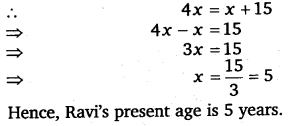Question 13.
A rational number is such that when you multiply it by$\frac { 5 }{ 2 }$ and add$\frac { 2 }{ 3 }$ to the product, you get$-\frac { 7 }{ 12 }$. What is the number ?

Solution.
Let the required number be x. Then,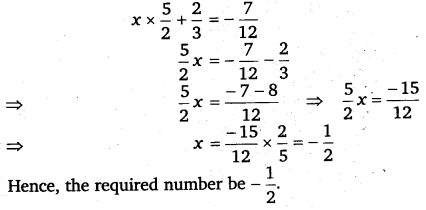Question 14.
Lakshmi is a cashier in a bank. She has currency notes of denominations ₹ 100, ₹ 50 and ₹ 10, respectively. The ratio of the number of these notes is 2 : 3 : 5. The total cash with Lakshmi is ₹ 4,00,000. How many notes of each denomination does she have ?

Solution.
Let notes of denominations ₹ 100, ₹ 50 and ₹ 10 be 2x, 3x and 5x respectively.Question 15.
I have a total oft 300 in coins of denomination ₹ 1, ₹ 2 and ₹ 5. The number of ₹ 2 coins is 3 times the number of ₹ 5 coins. The total number of coins is 160. How many coins of each denomination are with me ?

Solution.
Let the number of ₹ 5 coins be x. Then,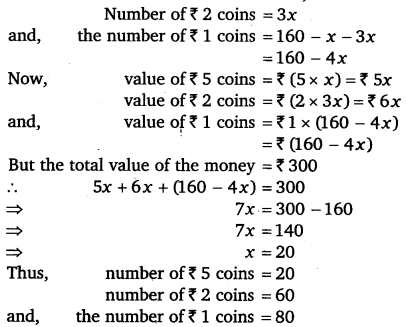Question 16.
The organisers of an essay competition decide that a winner in the competition gets a prize of ₹ 100 and a participant who does not win gets a prize of ₹ 25. The total prize money distributed is ₹ 3,000. Find the number of winners, if the total number of participants is 63.

Solution.
Let x be the number of the winners: So, the number of participants who does not win is (63 – x).#### Class 8 Maths NCERT Solutions for Chapter – 2 Linear Equations In One Variable Ex – 2.3

Lorem ipsum dolor sit amet, consectetur adipiscing elit. Phasellus cursus rutrum est nec suscipit. Ut et ultrices nisi. Vivamus id nisl ligula. Nulla sed iaculis ipsum.

Company Name